# 1 Introduction

The ref.ICAR package performs objective Bayesian analysis using a reference prior proposed by Keefe et al. (2019). This model provides an approach for modeling spatially correlated areal data, using an intrinsic conditional autoregressive (ICAR) component on a vector of spatial random effects with a reference prior for all model parameters. Ferreira et al. (2021) developed faster MCMC sampling for the ICAR model with reference prior, and Porter et al. (2023) developed objective Bayesian model selection based on fractional Bayes factors for the model.

# 2 Functions

ref.ICAR can be used to analyze areal data corresponding to a contiguous region, provided a shapefile or neighborhood matrix and data. The functions implemented by ref.ICAR are summarized below.

• shape.H() Takes a file path to a shapefile and forms the appropriate neighborhood matrix for analysis with the ICAR reference prior model.

• ref.MCMC() Implements the posterior sampling algorithm proposed by Keefe et al. (2019). Generates MCMC chains for parameter and regional inferences.

• ref.summary() Provides posterior inferences for the model parameters, $$\tau$$, $$\boldsymbol{\beta}$$, and $$\sigma^2$$. Includes posterior medians, highest posterior density (HPD) intervals, and acceptance rates for each parameter.

• reg.summary() Provides fitted posterior values and summaries for each subregion in the areal data set. Includes posterior medians and HPD intervals by region.

• ref.plot() Outputs trace plots for the model parameters, $$\tau$$, $$\boldsymbol{\beta}$$, and $$\sigma^2$$.

• ref.analysis() Performs analysis by sequentially implementing each of the functions above. This function produces plots and a list containing MCMC chains, parameter estimates, regional estimates, and sampling acceptance rates.

• probs.icar() Performs simultaneous, objective Bayesian model selection for covariates and spatial model structure for areal data. This function provides posterior model probabilities for all candidate ICAR models and OLMs.

# 3 ICAR Model Summary

The model implemented by ref.ICAR is summarized below. $\begin{equation} \mathbf{Y}= X \boldsymbol{\boldsymbol{\beta}}+\boldsymbol{\theta}+\boldsymbol{\phi} \end{equation}$

where

• $$\mathbf{Y}$$ is an $$n\times1$$ vector for the response variable, where $$n$$ corresponds to the number of regions in the shapefile. The current version of the package does not allow for missing data.

• $$X$$ is a matrix of covariates. This can include a vector $$\textbf{1}_n$$ for an intercept, and additional columns corresponding to quantitative predictors.

• $$\boldsymbol{\boldsymbol{\beta}}$$ is the $$p\times1$$ vector of fixed effect regression coefficients, where $$p$$ corresponds to the number of columns in $$X$$.

• $$\boldsymbol{\theta}$$ is an $$n\times1$$ vector of independent and normally distributed unstructured random effects defined with mean 0 and variance $$\sigma^2$$.

• $$\boldsymbol{\phi}$$ is an $$n\times1$$ vector of spatial random effects that is assigned an intrinsic CAR prior with the sum-zero constraint $$\sum_{i=1}^n \phi_i=0$$ (Keefe et al. 2018).

The model assumes a signal-to-noise ratio parameterization for the variance components of the random components of the model, so $$\sigma^2$$ and $$\tau$$ are used as below. $\boldsymbol{\phi} \sim \bigg(\textbf{0},\frac{\sigma^2}{\tau}\Sigma_{\phi}\bigg)$

The parameter $$\tau$$ controls the strength of spatial dependence, and given the neighborhood structure, $$\Sigma_{\phi}$$ is a fixed matrix. Specifically, $$\Sigma_{\phi}$$ is the Moore-Penrose inverse of $$H$$, where the neighborhood matrix $$H$$ is an $$n\times n$$ symmetric matrix constructed as follows. $\begin{equation} (H)_{ij} = \begin{cases} h_i & \text{if } i=j \\ -g_{ij} & \text{if } i\in N_j \\ 0 & \text{otherwise}, \end{cases} \end{equation}$

where $$g_{ij}=1$$ if subregions $$i$$ and $$j$$ are neighbors, $$g_{ij}=0$$ if subregions i and j are not neighbors,and $$h_i$$ is the number of neighbors of subregion $$i$$. Therefore, the neighborhood matrix $$H$$ is an $$n\times n$$ symmetric matrix where the diagonal elements correspond to the number of neighbors for each subregion in the data, and each off-diagonal element equals $$-1$$ if the corresponding subregions are neighbors.

Provided a path to a shapefile, the shape.H() function in ref.ICAR constructs $$H$$ as specified above, and checks for symmetry and contiguous regions (i.e. no islands) prior to analysis. The functions shape.H() and ref.analysis() requires a file path to a shapefile. If a user wants to analyze areal data without a corresponding shapefile (e.g. neuroimaging), they will need to construct $$H$$ as above and use this $$H$$ in ref.MCMC(). ref.plot(),ref.summary(), and reg.summary() can then be used with the MCMC chains obtained from ref.MCMC(). Additionally, if a user performs analysis without ref.analysis(), the regions corresponding to data values in $$X$$ and $$y$$ must match the region order in $$H$$; otherwise inferences will be matched to incorrect regions.

# 4 Example: Objective ICAR Inference

Consider an example of areal data over the contiguous United States. Figure 1 represents the average SAT scores reported in 1999 for each of the contiguous United States and Washington D.C. This example will explore these data and use the ref.ICAR package to fit a model to the response, Verbal SAT scores, considering spatial dependence and a single covariate, percent of eligible students that took the SAT in each state in 1999. This data was analyzed in Hierarchical Modeling and Analysis for Spatial Data (Banerjee et al. 2014). The data are available online at https://www.counterpointstat.com/hierarchical-modeling-and-analysis-for-spatial-data.html. We make it available in the ref.ICAR package with permission from the authors. The shapefile is found from http://www.arcgis.com/home/item.html?id=f7f805eb65eb4ab787a0a3e1116ca7e5.

These data and the accompanying shapefile are attached to the ref.ICAR package. The files can be loaded into R as shown below. The st_read() function from package sf is used to read the shapefile.

library(sf)

system.path <- system.file("extdata", "us.shape48.shp", package = "ref.ICAR",
mustWork = TRUE)
shp.layer <- gsub(".shp", "", basename(system.path))
shp.path <- dirname(system.path)

us.shape48 <- st_read(dsn = path.expand(shp.path), layer = shp.layer,
quiet = TRUE)

The SAT data can be loaded into R from ref.ICAR using read.table().

library(utils)

data.path <- system.file("extdata", "states-sats48.txt", package = "ref.ICAR",
mustWork = TRUE)

us.shape48$verbal <- sats48$VERBAL
us.shape48$percent <- sats48$PERCENT

Now that the shapefile and data are loaded, the observed data can be plotted as a choropleth map (Figure 1). This map illustrates the spatial dependence to be analyzed by the model. The Midwestern states and Utah exhibit the highest average SAT scores, and overall, neighboring states have similar average scores.

library(ggplot2)
library(classInt)
library(dplyr)

breaks_qt <- classIntervals(c(min(us.shape48$verbal) - 1e-05, us.shape48$verbal), n = 7, style = "quantile")

us.shape48_sf <- mutate(us.shape48, score_cat = cut(verbal, breaks_qt$brks)) ggplot(us.shape48_sf) + geom_sf(aes(fill = score_cat)) + scale_fill_brewer(palette = "OrRd") + labs(title = "Plot of observed \n verbal SAT scores") + theme_bw() + theme(axis.ticks.x = element_blank(), axis.text.x = element_blank(), axis.ticks.y = element_blank(), axis.text.y = element_blank(), axis.title = element_text(size = 25, face = "bold"), plot.title = element_text(face = "bold", size = 25, hjust = 0.5)) + guides(fill = guide_legend("Verbal score"))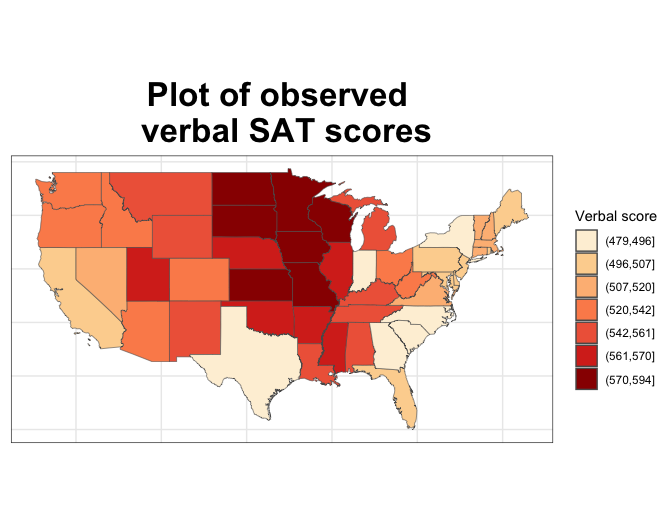Figure 1: Observed Verbal SAT Scores Similarly, the covariate, percent of eligible students taking the SAT, can be plotted over the contiguous United States. These data exhibit a seemingly inverse relationship to the SAT scores; lower percentages of students take the SAT in the Midwest. breaks_qt <- classIntervals(c(min(us.shape48$percent) - 1e-05,
us.shape48$percent), n = 7, style = "quantile") us.shape48_sf <- mutate(us.shape48, pct_cat = cut(percent, breaks_qt$brks))
ggplot(us.shape48_sf) + geom_sf(aes(fill = pct_cat)) + scale_fill_brewer(palette = "OrRd") +
labs(title = "Plot of observed \n percent SAT takers") +
theme_bw() + theme(axis.ticks.x = element_blank(), axis.text.x = element_blank(),
axis.ticks.y = element_blank(), axis.text.y = element_blank(),
axis.title = element_text(size = 25, face = "bold"), plot.title = element_text(face = "bold",
size = 25, hjust = 0.5)) + guides(fill = guide_legend("Percent taking"))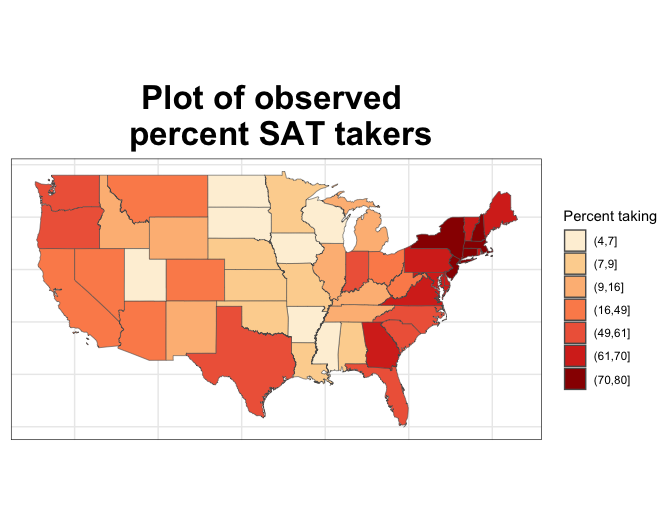Figure 2: Percent of eligible students taking the SAT

Employing the functions in ref.ICAR, the shape.H() function first takes the path to the shape file (obtained above), and returns a list of two objects. This list contains the neighborhood matrix, $$H$$ and a $$\texttt{SpatialPolygonsDataFrame}$$ object corresponding to the shapefile, to be used by the remaining functions.

library(ref.ICAR)
library(spdep)

shp.data <- shape.H(system.path)
## Reading layer us.shape48' from data source
##   /private/var/folders/zf/nsyh0fy90678j3c41vf9hg7r0000gn/T/RtmpPB1YHQ/Rinstc23461c7c44a/ref.ICAR/extdata'
##   using driver ESRI Shapefile'
## Simple feature collection with 49 features and 6 fields
## Geometry type: MULTIPOLYGON
## Dimension:     XY
## Bounding box:  xmin: -124.7328 ymin: 24.95638 xmax: -66.96927 ymax: 49.37173
## Geodetic CRS:  Clarke 1866
H <- shp.data$H class(shp.data$map)
##  "sf"         "data.frame"
length(shp.data$map) ##  7 The response and covariates, $$Y$$ and $$X$$ must be defined before fitting the model. The response, $$Y$$, is Verbal SAT scores. $$X$$ has two columns corresponding to an intercept and the predictor, percent of eligible students taking the SAT in 1999. Y <- sats48$VERBAL
x <- sats48$PERCENT X <- cbind(1, x) Then sampling can be performed using ref.MCMC(). The default starting values are used below, with MCMC iterations and burn-in larger than the default. The sampling for ref.MCMC() is based on developments by Ferreira et al. (2021), who express the spatial hierarchical model in the spectral domain to obtain the faster Spectral Gibbs Sampler (SGS). Previous versions of ref.ICAR implemented the Spectral Decomposition of the Precision (SDP) algorithm proposed by Keefe et al. (2019). See Ferreira et al. (2021) for an outline of the algorithm and computational comparisons. library(mvtnorm) set.seed(3456) ref.SAT <- ref.MCMC(y = Y, X = X, H = H, iters = 15000, burnin = 5000, verbose = FALSE) names(ref.SAT) ##  "MCMCchain" "tauc.MCMC" "sigma2.MCMC" "beta.MCMC" ##  "phi.MCMC" "accept.phi" "accept.sigma2" "accept.tauc" The object ref.SAT contains MCMC chains for each of the parameters in the model $$\mathbf{Y}= X \boldsymbol{\boldsymbol{\beta}}+\boldsymbol{\theta}+\boldsymbol{\phi}$$, using a signal-to-noise ratio parameterization. From these, the function ref.plot() creates trace plots for each parameter to visually confirm convergence. par(mfrow = c(2, 2)) ref.plot(ref.SAT$MCMCchain, X, burnin = 5000, num.reg = length(Y))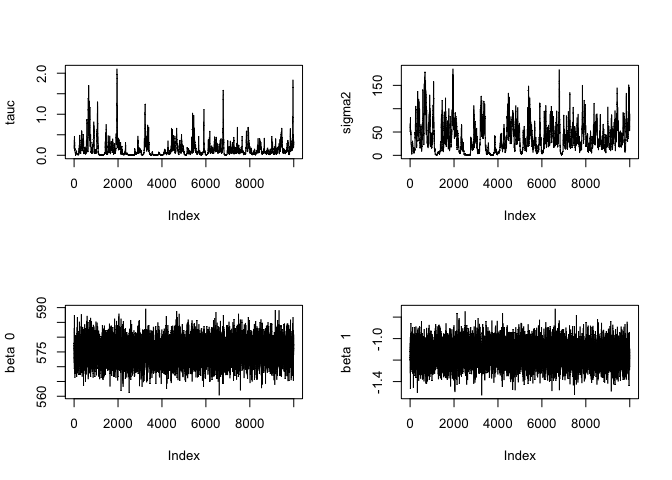The remaining components for the analysis are the functions for parameter and regional inferences. The function ref.summary() provides posterior medians and intervals for the model parameters $$\boldsymbol{\beta}$$, $$\tau$$, and $$\sigma^2$$. The function ref.summary() provides medians and Highest Posterior Density intervals for the fitted $$y$$ values for each subregion in the data.

library(coda)

summary.params <- ref.summary(MCMCchain = ref.SAT$MCMCchain, tauc.MCMC = ref.SAT$tauc.MCMC, sigma2.MCMC = ref.SAT$sigma2.MCMC, beta.MCMC = ref.SAT$beta.MCMC, phi.MCMC = ref.SAT$phi.MCMC, accept.phi = ref.SAT$accept.phi, accept.sigma2 = ref.SAT$accept.sigma2, accept.tauc = ref.SAT$accept.tauc, iters = 15000, burnin = 5000)

names(summary.params)
##  "beta.median"   "beta.hpd"      "tauc.median"   "tauc.hpd"
##  "sigma2.median" "sigma2.hpd"    "tauc.accept"   "sigma2.accept"
summary.params
## $beta.median ##  575.461382 -1.142777 ## ##$beta.hpd
##           lower       upper
## var1 568.028129 582.5163300
## var2  -1.334464  -0.9487258
##
## $tauc.median ##  0.08581395 ## ##$tauc.hpd
##        lower        upper
## 0.0003402439 0.5212723440
##
## $sigma2.median ##  31.2014 ## ##$sigma2.hpd
##      lower      upper
##  0.1604447 96.0084162
##
## $tauc.accept ##  0.3436667 ## ##$sigma2.accept
##  0.3436667

The posterior medians for $$\beta_0$$ and $$\beta_1$$ are 575.496 and -1.145, respectively. Additionally, the HPD interval for $$\beta_1$$ does not include $$0$$, which indicates that as the percent of eligible students taking the SAT increases, average Verbal SAT score tends to decrease. The $$\tau$$ median is 0.08, with HPD interval between 0.0014 and 0.5237.

summary.region <- reg.summary(ref.SAT$MCMCchain, X, Y, burnin = 5000) us.shape48$verbalfits <- summary.region$reg.medians breaks_qt <- classIntervals(c(min(us.shape48$verbalfits) - 1e-05,
us.shape48$verbalfits), n = 7, style = "quantile") us.shape48_sf <- mutate(us.shape48, reg_cat = cut(verbalfits, breaks_qt$brks))
ggplot(us.shape48_sf) + geom_sf(aes(fill = reg_cat)) + scale_fill_brewer(palette = "OrRd") +
labs(title = "Plot of fitted \n verbal SAT scores") + theme_bw() +
theme(axis.ticks.x = element_blank(), axis.text.x = element_blank(),
axis.ticks.y = element_blank(), axis.text.y = element_blank(),
axis.title = element_text(size = 25, face = "bold"),
plot.title = element_text(face = "bold", size = 25, hjust = 0.5)) +
guides(fill = guide_legend("Region medians"))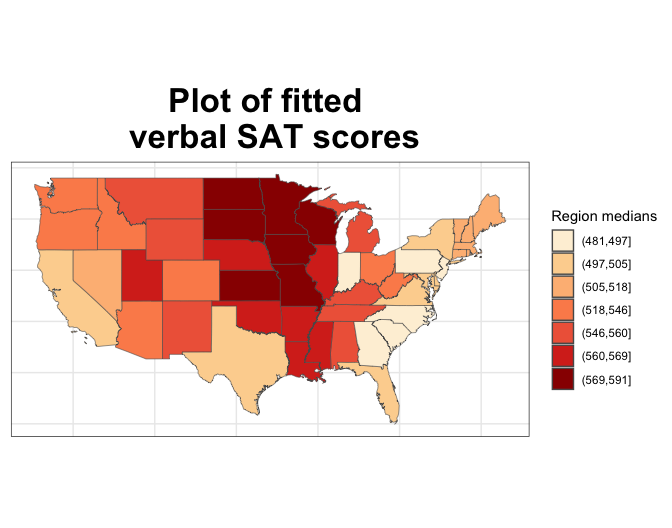Figure 3: Posterior Medians for Verbal SAT

Finally, the function ref.analysis() in ref.ICAR performs the entire reference analysis, including:

• Obtaining the neighborhood matrix, $$H$$

• Running the MCMC chains

• Producing parameter trace plots

• Producing parameter summaries

• Producing regional summaries

ref.analysis() requires the following user inputs: $$X$$, $$y$$, a path to a shapefile, a vector of region names corresponding to the values in $$X$$, and a vector of region names corresponding to the values in response $$y$$. The region names in each of $$X$$ and $$y$$ must match and are required because ref.analysis() reorders the data according to the region order in the shapefile. This ensures that the data values match to the correct entries in the neighborhood matrix $$H$$; otherwise analysis might map predicted values to incorrect regions. If the provided shapefile does not have a specified NAME column, the user will be asked to also provide a vector of names corresponding to the shapefile. This vector is called $$\texttt{shp.reg.names}$$ in the documentation and function arguments; the default value is NULL.

### The SAT scores and percent of students are already
### arranged by state alphabetically
x.reg.names <- us.shape48$NAME y.reg.names <- us.shape48$NAME

set.seed(3456)
par(mfrow = c(2, 2))
sat.analysis <- ref.analysis(system.path, X, Y, x.reg.names,
y.reg.names, shp.reg.names = NULL, iters = 15000, burnin = 5000,
verbose = FALSE, tauc.start = 0.1, beta.start = -1, sigma2.start = 0.1,
step.tauc = 0.5, step.sigma2 = 0.5)
## Reading layer us.shape48' from data source
##   /private/var/folders/zf/nsyh0fy90678j3c41vf9hg7r0000gn/T/RtmpPB1YHQ/Rinstc23461c7c44a/ref.ICAR/extdata'
##   using driver ESRI Shapefile'
## Simple feature collection with 49 features and 6 fields
## Geometry type: MULTIPOLYGON
## Dimension:     XY
## Bounding box:  xmin: -124.7328 ymin: 24.95638 xmax: -66.96927 ymax: 49.37173
## Geodetic CRS:  Clarke 1866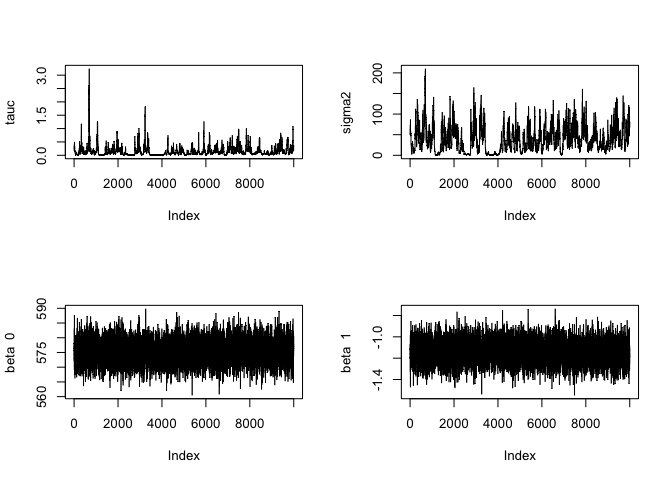names(sat.analysis)
##   "H"             "MCMC"          "beta.median"   "beta.hpd"
##   "tauc.median"   "tauc.hpd"      "sigma2.median" "sigma2.hpd"
##   "tauc.accept"   "sigma2.accept" "fit.dist"      "reg.medians"
##  "reg.hpd"

# 5 Example: Objective Model Selection for Areal Data

Porter et al. (2023) developed objective Bayesian model selection for simultaneous selection of covariates and spatial model structure for areal data. Since the joint reference prior on model parameters is improper (Keefe et al. 2019), fractional Bayes factor methodology is used to approximate Bayes factors and obtain valid posterior model probabilities for all candidate ICAR models and OLMs from the provided candidate covariates. See Porter et al. (2023) for the method details and simulation results, including the minimal training size for the fractional Bayes factor that is recommended for this approach. The following is a code example that uses case study data seen in Porter et al. (2023). The data is available with the spdep package, which is imported by ref.ICAR. The outcome of interest is the residential crime rate across the 49 neighborhoods of Columbus, Ohio. The five candidate predictors include average housing value, average household income, amount of open space in each neighborhood, the number of housing units without available plumbing, and distance from the Columbus business district. Reading the data into R using st_read(), creates an object, which includes the response variable and the candidate covariates.

library(pracma)
library(stats)
library(spdep)

# read in the data as contained in the spdep package
columbus <- st_read(system.file("shapes/columbus.shp", package = "spData"),
quiet = TRUE)

Similarly to the last example, we can plot the response variable, residential crime rate, over the geographic region to visualize which of the 49 neighborhoods have the highest observed crime rates.

breaks_qt <- classIntervals(c(min(columbus$CRIME) - 1e-05, columbus$CRIME),
n = 7, style = "quantile")

columbus_sf <- mutate(columbus, crime_cat = cut(CRIME, breaks_qt$brks)) ggplot(columbus_sf) + geom_sf(aes(fill = crime_cat)) + scale_fill_brewer(palette = "OrRd") + labs(title = "Plot of observed \n neighborhood crime rates") + theme_bw() + theme(axis.ticks.x = element_blank(), axis.text.x = element_blank(), axis.ticks.y = element_blank(), axis.text.y = element_blank(), axis.title = element_text(size = 25, face = "bold"), plot.title = element_text(face = "bold", size = 25, hjust = 0.5)) + guides(fill = guide_legend("Neighborhood \n crime rate"))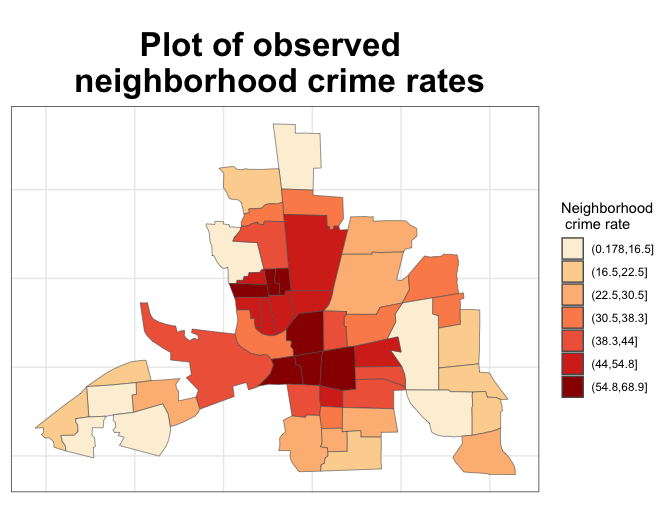Figure 4: Plot of observed crime rates for Columbus, OH neighborhoods Upon reading in the data, we can begin to fit each of the candidate models for the data based on combinations of the covariates and whether or not the model contains ICAR random effects. We consider all possible OLMs and ICAR models from the 5 candidate covariates, resulting in a model space of size $$2 \times 2^5=64$$. Each of the candidate ICAR models uses the same neighborhood matrix $$H$$, based on the 49 subregions, which we can define as follows. # create neighborhood matrix columbus.listw <- poly2nb(columbus) summary(columbus.listw) ## Neighbour list object: ## Number of regions: 49 ## Number of nonzero links: 236 ## Percentage nonzero weights: 9.829238 ## Average number of links: 4.816327 ## Link number distribution: ## ## 2 3 4 5 6 7 8 9 10 ## 5 9 12 5 9 3 4 1 1 ## 5 least connected regions: ## 1 6 42 46 47 with 2 links ## 1 most connected region: ## 20 with 10 links W <- nb2mat(columbus.listw, style = "B") Dmat <- diag(apply(W, 1, sum)) num.reg <- length(columbus$CRIME)

H <- Dmat - W
H <- (H + t(H))/2
rownames(H) <- NULL
isSymmetric(H)  # check that neighborhood matrix is symmetrix before proceeding
##  TRUE
# spectral quantities for use in model selection
H.spectral <- eigen(H, symmetric = TRUE)
Q <- H.spectral$vectors eigH <- H.spectral$values
phimat <- diag(1/sqrt(eigH[1:(num.reg - 1)]))
Sig_phi <- matrix(0, num.reg, num.reg)  #initialize
for (i in 1:(num.reg - 1)) {
total <- (1/(eigH[i])) * Q[, i] %*% t(Q[, i])
Sig_phi <- Sig_phi + total
}

# define response and design matrix
Y <- columbus$CRIME X <- cbind(1, columbus$HOVAL, columbus$INC, columbus$OPEN,
columbus$PLUMB, columbus$DISCBD)
b <- (ncol(X) + 1)/num.reg  # specify the minimal training size for this example

# perform model selection
columbus.select <- probs.icar(Y = Y, X = X, H = H, H.spectral = H.spectral,
Sig_phi = Sig_phi, b = b, verbose = FALSE)

# print the model with highest posterior model
# probability
columbus.select$probs.mat[which.max(columbus.select$probs.mat[,
1]), ]
##    model prob  model type                   model form
## 19  0.1193955 Independent Y ~ Intercept + X1 + X2 + X5
# print vector of posterior inclusion probabilities for
# each covariate
post.include.cov <- matrix(NA, nrow = 1, ncol = ncol(X) - 1)
labels <- c(rep(NA, ncol(X) - 1))
for (i in 1:(ncol(X) - 1)) {
labels[i] <- paste("X", i, sep = "")
}
colnames(post.include.cov) <- labels

for (j in 1:ncol(X) - 1) {
post.include.cov[, j] <- sum(columbus.select$probs.mat[grep(paste("X", j, sep = ""), columbus.select$probs.mat\$"model form"),
1])
}

post.include.cov
##             X1        X2        X3        X4        X5
## [1,] 0.7454222 0.9238743 0.3009956 0.4312049 0.9273156

As an extension to the objective Bayesian model selection for spatial ICAR models, the R package GLMMselect uses fractional Bayes factor methodology to simultaneously select fixed effects and random effects in Generalized Linear Mixed Models (GLMMs) where the covariance structure for the random effects is a product of a unknown scalar and a known semi-positive definite matrix. GLMMselect (https://CRAN.R-project.org/package=GLMMselect) can currently be used for model selection for Poisson and Bernoulli data, based on the methodology in Xu et al. (2023).